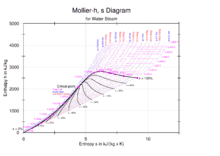# How do I find an enthelpy value if my temp. is below 50 deg. celcius?

bakharuddin
I want to design a curding machine (Milk to Cheese) with an open system that operate at isobaric process (contant pressure),
P = 1 atm = 1,... bar
the range of temperature is = 25-75 0C
can i find an enthalpy of water from Mollier Diagram if my temp. is 25 to 75 degree celcius ? how ?

from my view, i must separates water properties from milk to make curds.
so i use mollier diagram but still confuse about it.#### Attachments

Mentor
What is the heat capacity of water?

•bakharuddin
bakharuddin
What is the heat capacity of water?
the amount of heat needed to raise its temperature a certain amount. One of water's most significant properties is that it takes a lot of heat to it to make it get hot. Precisely, water has to absorb 4,184 Joules of heat for the temperature of one kilogram of water to increase 1 degree celsius (°C)

Mentor
the amount of heat needed to raise its temperature a certain amount. One of water's most significant properties is that it takes a lot of heat to it to make it get hot. Precisely, water has to absorb 4,184 Joules of heat for the temperature of one kilogram of water to increase 1 degree celsius (°C)
So, why can't you use this to get the enthalpy of water at 50 C (consistent with the Mollier diagram reference state)?

•bakharuddin
bakharuddin
So, why can't you use this to get the enthalpy of water at 50 C (consistent with the Mollier diagram reference state)?

is there any equation to help me maybe ? still confuse to use this diagramMentor
is there any equation to help me maybe ? still confuse to use this diagramWhy are you using a Mollier diagram? Why aren't you using the Steam Tables? The change in enthalpy with respect to temperature is $$\Delta H=mC_p\Delta t$$assuming no change of phase.

•russ_watters
bakharuddin
Why are you using a Mollier diagram? Why aren't you using the Steam Tables? The change in enthalpy with respect to temperature is $$\Delta H=mC_p\Delta t$$assuming no change of phase.

because from mollier diagram there is an x value which is a moisture of water parameter, and i think is a must factor to include.
am i wrong ? or is it more simple using steam table to get the enthalpy ?

Mentor
because from mollier diagram there is an x value which is a moisture of water parameter, and i think is a must factor to include.
am i wrong ? or is it more simple using steam table to get the enthalpy ?
The moisture parameter gives the fraction that’s vapor, but, in your case, you have purely a liquid stream, right? It is, of course, simpler to use the steam tables(or even the equation), and it’s more accurate than reading off a graph. You seem to need to review thetmo to get the basic concepts down pat.

•bakharuddin
bakharuddin
The moisture parameter gives the fraction that’s vapor, but, in your case, you have purely a liquid stream, right? It is, of course, simpler to use the steam tables(or even the equation), and it’s more accurate than reading off a graph. You seem to need to review thetmo to get the basic concepts down pat.

Okay ill try it using the steam table.
Thanks you sir, i really appreciate that. glad someone can help here•Chestermiller